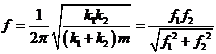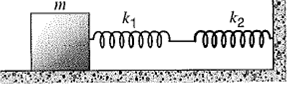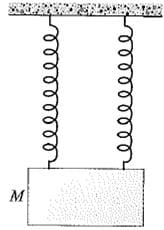# Solved Examples: Oscillations Notes | Study Mock Test Series for JEE Main & Advanced 2022 - JEE

## JEE: Solved Examples: Oscillations Notes | Study Mock Test Series for JEE Main & Advanced 2022 - JEE

The document Solved Examples: Oscillations Notes | Study Mock Test Series for JEE Main & Advanced 2022 - JEE is a part of the JEE Course Mock Test Series for JEE Main & Advanced 2022.
All you need of JEE at this link: JEE

Question 1: Two springs are joined and connected to a block of mass m as shown in below figure. The surfaces are frictionless. If the springs are separately have force constants k1 and k2, show that the frequency of oscillation of the block isWhere f1 and f2 are the frequencies at which the block would oscillate if connected only to spring 1 or spring 2. (The electrical along of this system is a parallel combination of two capacitors.)Concept:
In simple harmonic motion,
(a) The time period (T) of vibrations varies inversely as the square root of the force constant (k) of the spring.
(b) The time period (T) of vibrations varies directly as the square root of the mass (m) of body attached to the string.
Thus the time period of an object of mass M on a spring executes a simple harmonic motion is given by,
T = 2π √m/k
So frequency f of the oscillation would be,
f = 1/T
= 1/(2π √m/k)    (Since, T = 2π √m/k)
= 1/2π √k/m
In an oscillating system, restoring force F is defined as,
F = k
Here k is the spring constant and x is the displacement of the particle from its mean position.
So the spring constant k will be,
k = F/x
Solution:
The restoring force Facting on the mass m due to the spring having force constant k1 will be ,F1 = k1x1
Here x1 is the elongation.
The restoring force F2 acting on the mass m due to the spring having force constant k2 will be, F2 = k2x2
Here x2 is the elongation.
The tension in the two springs will be same.
So, k1x1 = k2x2
But the total extension is x1 + x2.
Thus the effective spring constant k of the combination will be,
k = F/x
= F/(x1+x2)
= 1/ [x1/F + x2/F ]
= 1/ [1/k1 +1/k2]
= k1k2/ k1+ k2
To find out the frequency of oscillation f, substitute k1k2/ k1+ k2 for the spring constant k in the equation f = 1/2π √k/m, we get,
f = 1/2π √k/m
= 1/2π √k1k2/(k1+k2)m
= 1/2π √1/m/k1 +m/k2
=1/2π √1/1/w12 + 1/w22( Since, w12 = k1/m and w22 = k2/m )
=1/2π √ w12w22/w12 +w22
= f1f2/√f12+f22     (Since, w1 = 2πf1 and w2 = 2πf2)
From the above observation we conclude that, the frequency of oscillation of the block will be f1f2/√f12+f2.

Question 2: A massless spring of force constant 3.60 N/cm is cut into halves. (a) What is the force constant of each half? (b)The two halves, suspended separately, support a block of mass M (see below figure). The system vibrates at a frequency of 2.87 Hz. Find the value of the mass M.Concept:
In simple harmonic motion,
(c) The time period (T) of vibrations varies inversely as the square root of the force constant (k) of the spring.
(d) The time period (T) of vibrations varies directly as the square root of the mass (m) of body attached to the string.
Thus the time period of an object of mass M on a spring executes a simple harmonic motion is given by,
T = 2π √m/k
So frequency f of the oscillation would be,
f = 1/T
= 1/(2π √m/k)
= 1/2π √k/m
In an oscillating system, restoring force F is defined as,
F = kx
Here k is the force constant and x is the displacement of the particle from its mean position.
So, k = F/x
Solution:
(a) When a spring is stretched the tension is the same everywhere in the spring. The stretching, however, is distributed over the entire length of the spring. Thus the relative amount of stretch is proportional to the length of the spring under consideration.
As the spring cut into halves, so the extension will be half.
To obtain the new force constant k', substitute x/2 in the equation k = F/x,
k' = F/(x/2)
=2F/x
= 2k        (Since, k = F/x)
To find out the new force constant k' for each half of the spring, substitute 3.60 N/cm for k in the equation k'= 2k,
k'= 2k
= 2(3.60 N/cm)
=7.20 N/cm
From the above observation we conclude that, the force constant of each half will be 7.20 N/cm.
(b) As the two halves, suspended separately, support a block of mass M, so we can view this configuration as each spring is holding one-half of the total mass.
Substitute M/2 for m in the equation f = 1/2π √k/m,
f = 1/2π √k/m
= 1/2π √k/(M/2)
So, k/(M/2) = 4 π2f 2
2k/M = 4 π2f 2
Or, M = 2k/4 π2f 2
To obtain the value of the mass M, substitute 7.20 N/cm for the k and 2.87 Hz for f in the equation M = 2k/4 π2f 2,
M = 2k/4 π2f 2
= 2(7.20 N/cm)/4 π(2.87 Hz)2
= 2(7.20 N/cm (1 cm/10-2 m))/4 π2 (2.87 Hz (1/1s/1 Hz))2
= 2(720 N/m)/4 π2 (2.87 s-1)2
= 4.43 N. s2/m
= (4.43 N. s2/m) [(1 kg.m/s2) /1 N]
= 4.43 kg
From the above observation we conclude that, value of the mass M would be 4.43 kg.

Question 3: A physical pendulum consists of a meter stick that is pivoted at a small hole drilled through the stick a distance x from the 50.0-cm mark. The period of oscillation is observed to be 2.50 s. Find the distance x.
Concept:
The period T of a physical pendulum is given by,
T = 2π√I/Mgd
Here, rotational inertia of the body about an axis through the pivot is I, mass of the body is M, acceleration due to gravity is gand distance from the pivot to the center of mass is d.
From the above equation, the rotational inertia will be,
I = T2Mgd/4π2
Solution: As the physical pendulum consists of a meter stick that is pivoted at a small hole drilled through the stick a distance x from the 50.0-cm mark, so we have to write x in place of d in the equation I = T2Mgd/4π2,
I = T2Mgx/4π2…… (1)
Since the hole is drilled off center through the stick a distance x, we have to apply principle axis theorem to obtain the rotational inertia of the pendulum.
Applying principle axis theorem, the rotational inertia I of the pendulum will be,
I = 1/12 ML2 + Mx2…… (2)
Here, mass of the pendulum is M and length is L.
Equating equation (1) and (2) we get,
1/12 ML2 + Mx2 = T2Mgx/4π2
Or, 1/12 L2 + x2 = T2gx/4π2
To obtain the value of distance x, substitute 1.00 m for L, 2.50 s for period T and 9.81 m/s2 for acceleration due to gravity g in the equation 1/12 L2 + x2 = T2gx/4π2, we get, 1/12
L2 + x2 = T2gx/4π2,
1/12 L+ x2 = T2gx/4π2
1/12 (1.00 m)2 + x2 = (2.50 s)2 (9.81 m/s2) x/4(3.14)2
x2 – (1.55 m) x + (8.33×10-2 m2) = 0
Or, x = - (-1.55) ±√(-1.55)2 -4(1)( 8.33×10-2)/2
= 1.49 m or 0.0557 m
From the above observation we conclude that, the value of distance x would be 1.49 m or 0.0557 m.

The document Solved Examples: Oscillations Notes | Study Mock Test Series for JEE Main & Advanced 2022 - JEE is a part of the JEE Course Mock Test Series for JEE Main & Advanced 2022.
All you need of JEE at this link: JEEUse Code STAYHOME200 and get INR 200 additional OFF

## Mock Test Series for JEE Main & Advanced 2022

2 videos|258 docs|160 tests

Track your progress, build streaks, highlight & save important lessons and more!

,

,

,

,

,

,

,

,

,

,

,

,

,

,

,

,

,

,

,

,

,

;